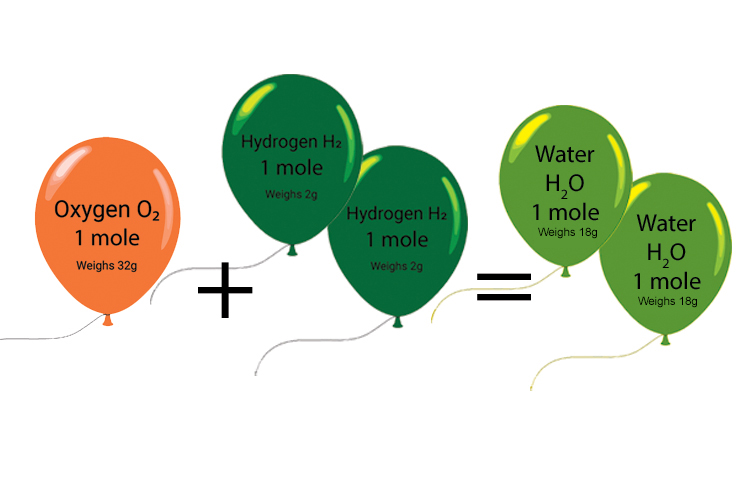# Examples of moles of gases

### Example 1

Calculate the volume of carbon dioxide gas, CO2, occupied by 5 moles of the molecule.

First work out the volume of 1 mole of carbon dioxide molecules

1 mole of CO2 molecules occupies 24 litres (RTP)

Therefore 5 moles of CO2 molecules = 5 x 24 litres = 120 litres.

### Example 2

Calculate the volume of helium gas, He, occupied by 10 moles of the atom.

First work out the volume of 1 mole of helium

1 mole of He atoms occupies 24 litres (RTP)

Therefore 10 moles of He atoms = 10 x 24 litres = 240 litres.

### Example 3

Calculate the volume of oxygen gas, O2, occupied by 0.75 moles of the molecule.

First work out the volume of 1 mole of oxygen molecules

1 mole of O2 molecules occupies 24 litres (RTP)

Therefore 0.75 moles of O2 molecules = 0.75 x 24 litres = 18 litres.

### Example 4

Calculate the volume of hydrogen gas, H2, occupied by 8.3 million moles of the molecule.

First work out the volume of 1 mole of hydrogen molecules

1 mole of H2 molecules occupies 24 litres (RTP)

Therefore 8.3 million moles of H2 molecules = 8.3 million x 24 litres = 199,200,000 litres.This was the volume of hydrogen in the Hindenburg Air Ship in the 1930s (about 200 million litres!)

### Example 5

1 mole of O2 molecules reacts with 2 moles of H2 molecules to produce 2 moles of H2O molecules. What is the weight of hydrogen and oxygen used up and what weight of water is produced?

1 mole of O2 molecules

Oxygen = There are 16 protons and neutrons in oxygen, each weighing 1
6 x 1023

1 mole oxygen = 6 x 1023   x16
6 x 1023
= 16g

But there are two oxygen atoms in O2. 16 x 2 = 32g

2 moles of H2 molecules

Hydrogen = There is one proton in hydrogen, weighing 1
6 x 1023
g

2 moles of hydrogen = 2 x 6 x 1023 x 1
6 x 1023
= 2g

But there are two hydrogen atoms in H2. Therefore 2 x 2 = 4g

32g oxygen gas + 4g hydrogen gas = 36g water produced.